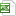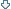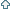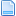Subjects(version: 855)CodeTitleSemester Hours per week, examination DepartmentAM413001 Fourier Transform winter winter s.:2/2 C+Ex [HT] 413AM413004 Multivariate data analysis summer summer s.:2/2 C+Ex [HT] 413AM413007 Mathematics for chemical engineers winter winter s.:2/2 C+Ex [HT] 413AM413010 Numerical algorithms winter winter s.:2/2 C+Ex [HT] 413AP413001 Graph Theory and Applications both 3/0 other [HT] 413AP413002 Numerical Linear Algebra both 3/0 other [HT] 413AP413003 Numerical Methods for Engineering both 3/0 other [HT] 413AP413004 Deterministic and stochastic discrete systems both 3/0 other [HT] 413AP413005 Numerical methods of Analysis of Non-linear Dynamical Models both 3/0 other [HT] 413AP413006 Non-linear Optimalization both 3/0 other [HT] 413AP413007 Dynamical Systems both 3/0 other [HT] 413AP413008 Fourier Transform both 3/0 other [HT] 413AP413009 Advanced Methods of Applied Mathematics both 3/0 other [HT] 413B413001 Mathematics A both 3/4 C+Ex [HT] 413B413002 Mathematics B both 3/3 C+Ex [HT] 413B413003 Applied Statistics both 1/2 C+Ex [HT] 413B413005 Computer Algebra System Maple summer summer s.:0/2 MC [HT] 413B413007 Secondary School Mathemetics Repetition winter winter s.:0/2 MC [HT] 413B413008 Elementary Mathematics summer summer s.:2/2 C+Ex [HT] 413B413009 Introduction to Mathematical Optimization summer summer s.:2/2 C+Ex [HT] 413B413010 Financial Mathematics summer summer s.:2/1 MC [HT] 413B413011 Discrete Mathematics summer summer s.:2/1 MC [HT] 413B413012 Selected chapters in Mathematics summer summer s.:2/2 C+Ex [HT] 413B413013 Mathematics A: Seminar winter winter s.:0/2 C [HT] 413D413002 Numerical Methods for Engineering winter winter s.:0/0 other [HT] 413D413004 Discrete Optimalization winter winter s.:0/0 other [HT] 413D413006 Numerical methods of Analysis of Non-linear Dynamical Models I, II winter winter s.:0/0 other [HT] 413D413007 Non-linear Optimalization both 0/0 other [HT] 413D413011 Dynamical Systems I, II winter winter s.:0/0 other [HT] 413D413012 Graph Theory and Applications winter winter s.:0/0 other [HT] 413D413018 Numerical Linear Algebra winter winter s.:0/0 other [HT] 413D413026 Fourier Transform winter winter s.:0/0 other [HT] 413D413027 Informatics in thermodynamics both 0/0 other [HT] 413D413028 Advanced Methods of Applied Mathematics summer summer s.:0/0 other [HT] 413M413001 Fourier Transform winter winter s.:2/2 C+Ex [HT] 413M413002 Systems of Ordinary Differential Equations (ODE) winter winter s.:2/2 C+Ex [HT] 413M413003 Methods of Applied Mathematics summer summer s.:2/1 C+Ex [HT] 413M413004 Multivariate data analysis summer summer s.:2/2 C+Ex [HT] 413M413006 Methods of Analysis of Non-linear Dynamical Models summer summer s.:2/2 C+Ex [HT] 413M413007 Mathematics for chemical engineers winter winter s.:2/2 C+Ex [HT] 413M413008 Partial Differential Equations (PDE) summer summer s.:3/0 Ex [HT] 413M413009 Mathematical Modeling and Optimal Control summer summer s.:2/1 C+Ex [HT] 413N413003 Mathematics II both 3/3 C+Ex [HT] 413N413003A Mathematics II winter winter s.:3/3 C+Ex [HT] 413N413004 Applied Statistics summer summer s.:1/2 C+Ex [HT] 413N413004A Applied Statistics winter winter s.:1/2 C+Ex [HT] 413N413005 Numerical Methods both 3/2 C+Ex [HT] 413N413007 Systems of Ordinary Differential Equations (ODE) winter winter s.:2/2 C+Ex [HT] 413N413008A Applied Mathematics summer summer s.:2/2 C+Ex [HT] 413N413009 Introduction to Mathematical Optimization summer summer s.:2/2 C+Ex [HT] 413N413011 Optimization of Engineering Processes winter winter s.:2/2 C+Ex [HT] 413N413012 Methods of Analysis of Non-linear Dynamical Models summer summer s.:2/2 C+Ex [HT] 413N413013 Methods of Applied Mathematics summer summer s.:2/1 C+Ex [HT] 413N413014 Partial Differential Equations (PDE) summer summer s.:3/0 Ex [HT] 413N413015 Mathematical Fundamentals of Optimal Control and Games Theory winter winter s.:2/1 C+Ex [HT] 413N413017 Financial Mathematics summer summer s.:2/1 MC [HT] 413N413018 Discrete Mathematics winter winter s.:2/1 MC [HT] 413N413019 Elementary Mathematics summer summer s.:2/2 C+Ex [HT] 413N413021 Mathematics B both 3/3 C+Ex [HT] 413N413022 Mathematics I both 3/4 C+Ex [HT] 413N413032 Mathematics for chemical engineers winter winter s.:2/2 C+Ex [HT] 413N413033 Mathematics for Quantum Chemistry summer summer s.:2/2 C+Ex [HT] 413N413038 Numerical algorithms winter winter s.:3/2 C+Ex [HT] 413N413038A Numerical algorithms summer summer s.:3/2 C+Ex [HT] 413N413039 Fourier transform for Bc. students winter winter s.:2/2 C+Ex [HT] 413N413040 Multivariate data analysis summer summer s.:2/2 C+Ex [HT] 413P413001 Graph Theory and Applications both 3/0 other [HT] 413P413002 Numerical Linear Algebra both 3/0 other [HT] 413P413003 Numerical Methods for Engineering both 3/0 other [HT] 413P413004 Deterministic and stochastic discrete systems both 3/0 other [HT] 413P413005 Numerical methods of Analysis of Non-linear Dynamical Models both 3/0 other [HT] 413P413006 Non-linear Optimalization both 3/0 other [HT] 413P413007 Dynamical Systems both 3/0 other [HT] 413P413008 Fourier Transform both 3/0 other [HT] 413P413009 Advanced Methods of Applied Mathematics both 3/0 other [HT] 413S413003 Mathematics II summer summer s.:3/3 C+Ex [HT] 413S413004 Applied Statistics summer summer s.:1/2 C+Ex [HT] 413S413005 Numerical Methods winter winter s.:3/2 C+Ex [HT] 413S413022 Mathematics I both 3/4 C+Ex [HT] 413S413032 Mathematics for chemical engineers winter winter s.:2/2 Ex [HT] 413